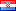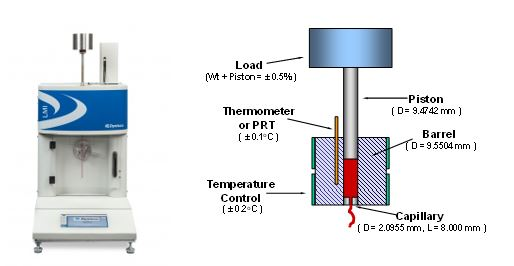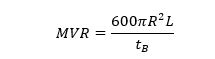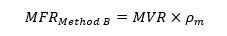# Rheological articles

## Different Test Methods for Measuring Melt Flow Rate of Plastic Materials Using a Melt Indexer

A flow rate test is used to determine the melt flow rate (MFR) of a thermoplastic material. According to ASDTM 1238, “Method A” test involves collecting extrudate from the die over a given period of time and under specified conditions of temperature and load.  Mass melt flow rate is then calculated based on the mass of the extrudate(s) as equation below

#### 06.19.2019

A flow rate test is used to determine the melt flow rate (MFR) of a thermoplastic material. According to ASDTM 1238, “Method A” test involves collecting extrudate from the die over a given period of time and under specified conditions of temperature and load.  Mass melt flow rate is then calculated based on the mass of the extrudate(s) as equation below

where  is the mass in grams of material collected over time . The MFR value has units g/10min (grams extruded in 10 minutes). “Procedure A” is a completely manual procedure and is sometimes called the “cut-n-weight” method.Method B test on the other hand is an assessment of a material`s flow characteristics based on the volumetric displacement rather than weight of extrudate with time as in Method A. Unlike Method A, no cutting and weighing of the extrudate is required to perform a Method B test. Results from Method B test can be expressed directly as Melt Volume-flow Rate (MVR) in cc/10min. To relate the results of a Method B run back to Method A, the apparent melt density calculated from Method A to B conversion is required. Method B test is a “no-cuts” test. As a result, it is simpler to run and more precise for routine analysis.

The MVR from Method B is calculated based on the volumetric displacement of the extrudate(s) as equation belowwhere R is barrel radios (R= 0.477cm) tb is the time of the piston travel for distance of L(flag length).  L equals to 0.635 cm based on ASTM 1238. The value of “600” has been taken into account in order to make the unit of MVR as cc/10min.

Multiplying the calculated MVR by melt density of the material, gives the value of the MFR (g/10min) as equation belowwhere Pm  (g/cm3) is polymer`s apparent melt density at the test temperature. Please note that melt density of the polymer is the function temperature. Also, fillers, reinforcing agent. etc. tend to increase the melt density of the material.

This test method is useful for quality control of materials from different processes or supplier lots. MFR is an empirically defined parameter which is influenced by the physical properties and molecular structure of the polymer materials such as viscosity, molecular weight, molecular weight distribution, moisture in material, degradation, crosslinking, or additives. Some of other applications of this test is determination of some polymer characteristics such as shear thinning of the polymer melt, extrudate swell, thermal stability, and intrinsic viscosity.